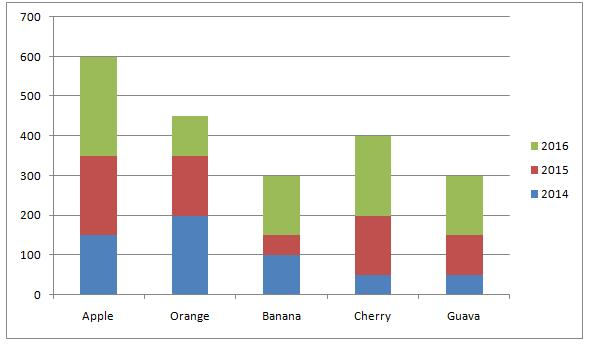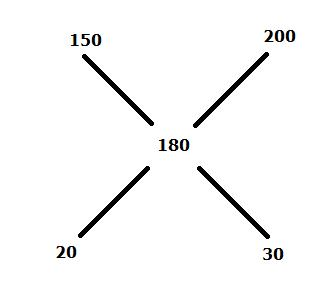# SBI PO Prelims Quantitative Aptitude (Day-19)

Dear Aspirants, Our IBPS Guide team is providing new series of Quantitative Aptitude Questions for SBI PO 2020 so the aspirants can practice it on a daily basis. These questions are framed by our skilled experts after understanding your needs thoroughly. Aspirants can practice these new series questions daily to familiarize with the exact exam pattern and make your preparation effective.

Start Quiz

Ensure Your Ability Before the ExamTake SBI PO 2020 Prelims Free Mock            Test

Simplification

Direction (01-05): What should be come in the place of question mark in the following questions?

1) 132  – √289 + 18 % of 550 + √2401 = ?

A) 400

B) 200

C) 300

D) 100

E) None of these

2) 80 % of 270 + 12.5 % of 1536 – 12*9 – 180 = ?

A) 120

B) 160

C) 110

D) 130

E) None of these

3) 150% of 120 + √1521 * 3 – 497 + 374 = ?

A) 174

B) 164

C) 184

D) 194

E) None of these

4) (0.7)64 ÷ (0.343)20 * 0.49 = (0.7)?

A) 6

B) 8

C) 4

D) 12

E) None of these

5) (70)– (64)+ √6561 + 1331 ÷ 11 + ?=1010

A) 8

B) 4

C) 6

D) 7

E) None of these

Data Interpretation

Direction (06-10): Study the following information carefully and answer the given questions:

The given bar graph shows the price (per kg) of five different fruits.6) The average price of Cherry in all the years together is approximately what percent of the average price of Orange in all the years together?

A) 89%

B) 93%

C) 83%

D) 87%

E) None of these

7) Suresh buys 10kg of apple and 25kg of Guava in the year 2014. He sells all these fruits in 2016 at the cost price in that year. Find the approximate profit percentage he got?

A) 127%

B) 123%

C) 124%

D) 131%

E) None of these

8) A shopkeeper sells the Orange at the rate in the year 2015 to the customers. 25% of the profit would be earned, if the selling price of the orange was decrease by 20%, then how much profit was earned on every kg of orange sold?

A) Rs.54

B) Rs.63

C) Rs.45

D) Rs.72

E) None of these

9) A shopkeeper mixes the Cherry in 2016 and 2015. He sells mixture at a profit of 25%. Total mixture is 450kg.If cost price of the mixture is Rs.180 per kg, find the difference between the total cost price of highest cost price and total selling price of the mixture?

A) Rs.47250

B) Rs.43750

C) Rs.39750

D) Rs.36250

E) None of these

10) What is the ratio of the average price of all the fruits in 2014 and the average price of all the fruits in 2016?

A) 11:17

B) 13:18

C) 9:19

D) 12:19

E) None of these

169-17+99+49=?

300=?

216 + 192 – 108 – 180 = ?

120=?

180 + 117 – 497 + 374 = ?

174=?

(0.7)64 ÷ ((0.7)3)20 * 0.7= (0.7)?

(0.7)64-60+2 = (0.7)?

? = 6

4900-4096+81+121+?=1010

4=?

Average price of cherry = (50+150+200) / 3 = 400/3

Average price of Orange = (200+150+100) / 3 = 450/3

Required percentage = ((400/3) / (450/3))*100 = 88.889 = 89%

Total price in 2014 = 10*150+25*50 = 2750

Total price in 2016 = 10*250+25*150 = 6250

Required percentage = ((6250-2750) / 2750) * 100 = 127%

SP=150 * (80/100 ) = 120

Cost price= (120*100) /125=96

Profit = 150-96 = Rs.54=2:3

Highest cost cherry = (3/5) * (450 * 200) = Rs.54000

Selling the mixture = (450*180*125)/100 = 101250

Required Difference= 101250 – 54000 = 47250

The average price of all the fruits in 2014 = (150+200+100+50+50)/5 = 110

The average price of all the fruits in 2016 =(250+100+150+200+150)/5= 170

Required ratio =110:170=11:17

 Check Here to View SBI PO Prelims 2020 Quantitative Aptitude Questions Day – 18 Day – 17 Day – 16 Click Here for SBI Clerk 2020 – Detailed Exam Notification